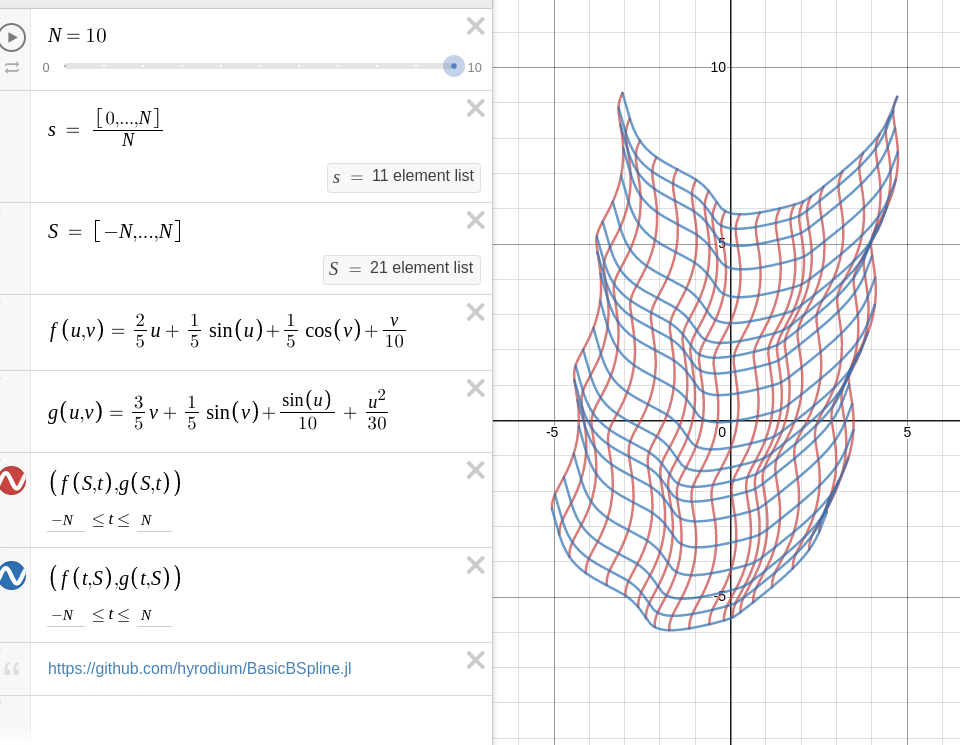# Fitting with B-spline manifold

The following functions such as fittingcontolpoints were provided from BasicBSpline.jl before v0.9.0. From BasicBSpline v0.9.0, these functions are moved to BasicBSplineFitting.

Fitting with least squares method.

BasicBSplineFitting.fittingcontrolpointsFunction

Fitting controlpoints with least squares method.

fittingcontrolpoints(func, Ps::Tuple)

This function will calculate $\bm{a}_i$ to minimize the following integral.

$$$\int_I \left\|f(t)-\sum_i B_{(i,p,k)}(t) \bm{a}_i\right\|^2 dt$$$

Similarly, for the two-dimensional case, minimize the following integral.

$$$\int_{I^1 \times I^2} \left\|f(t^1, t^2)-\sum_{i,j} B_{(i,p^1,k^1)}(t^1)B_{(j,p^2,k^2)}(t^2) \bm{a}_{ij}\right\|^2 dt^1dt^2$$$

Currently, this function supports up to three dimensions.

Examples

julia> f(t) = SVector(cos(t),sin(t),t);

julia> P = BSplineSpace{3}(KnotVector(range(0,2π,30)) + 3*KnotVector([0,2π]));

julia> a = fittingcontrolpoints(f, P);

julia> M = BSplineManifold(a, P);

julia> norm(M(1) - f(1)) < 1e-5
true
source
p1 = 2
p2 = 2
k1 = KnotVector(-10:10)+p1*KnotVector([-10,10])
k2 = KnotVector(-10:10)+p2*KnotVector([-10,10])
P1 = BSplineSpace{p1}(k1)
P2 = BSplineSpace{p2}(k2)

f(u1, u2) = SVector(2u1 + sin(u1) + cos(u2) + u2 / 2, 3u2 + sin(u2) + sin(u1) / 2 + u1^2 / 6) / 5

a = fittingcontrolpoints(f, (P1, P2))
M = BSplineManifold(a, (P1, P2))
save_png("fitting.png", M, unitlength=50, xlims=(-10,10), ylims=(-10,10))Try on Desmos graphing graphing calculator!